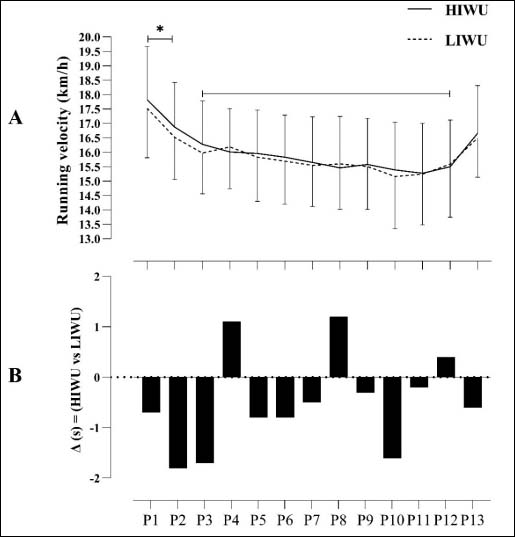Figure 3. A) Average running speed (km/h) in the 13 splits (mean ± standard deviation). B) Delta of the average times in seconds in the 13 split times (HIWU vs. LIWU). A) Average running speed (km/h) in the 13 splits (mean ± standard deviation): P1 = 200 m, P2 = 600 m, P3 = 1000 m, P4 = 1400 m, P5 = 1800 m, P6 = 2200 m, P7 = 2600 m, P8 = 3000 m, P9 = 3400 m, P10 = 3800 m, P11 = 4200 m, P12 = 4600 m and P13 = 5000 m. *p < 0.05 vs. the interval P3-P12. B) Delta of the average times in seconds in the 13 split times (HIWU vs. LIWU).